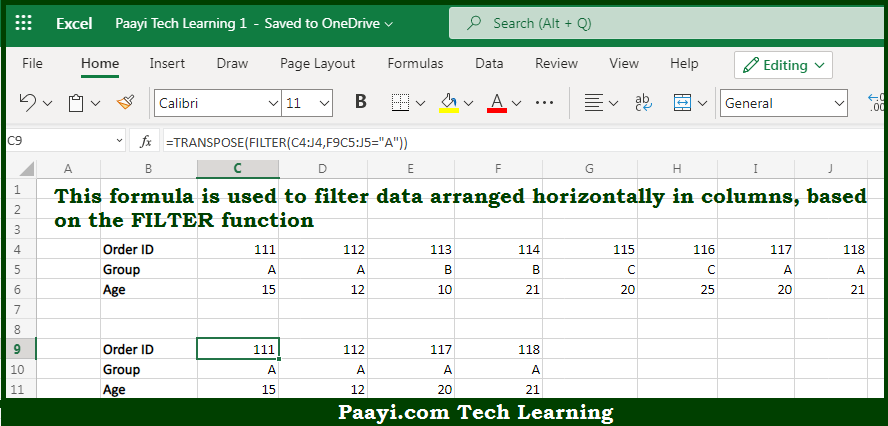# Learn How to Filter Horizontal Data in Microsoft Excel

Written by | 0 Comments | 545 Views

In this article, you will learn how to evaluate things in Dynamic Arrays with formulas in Microsoft Excel using a single/combination(s) of functions. You will also know How to Filter Horizontal Data and see the generic formula.

Learn How to Filter Horizontal Data in Microsoft Excel

The main purpose of this formula is to filter data arranged horizontally in columns. Here we will learn how to filter horizontal data in the given range in the workbook in Microsoft Excel. That implies, with the help of a formula based on the FILTER function you can able to filter data arranged horizontally in columns. So, with the help of this formula, you can able to filter horizontal data in the given range in the workbook in Microsoft Excel.

General Formula to Filter Horizontal Data

=FILTER(data,logic)

The Explanation for the Filter Horizontal DataSo we know that with the help of the given formula above you can able to filter data arranged horizontally in columns. Here we will learn how to filter horizontal data in the given range in the workbook in Microsoft Excel. As we know that the  FILTER function is utilized to extract data arranged vertically in rows or horizontally in columns. Besides that, the FILTER function will return the matching data in the same orientation.  Moreover, we don't need a special setup to perform this. So, with the help of this formula, you can able to filter data arranged horizontally in columns. Here we will learn how to filter horizontal data in the given range in the workbook in Microsoft Excel.